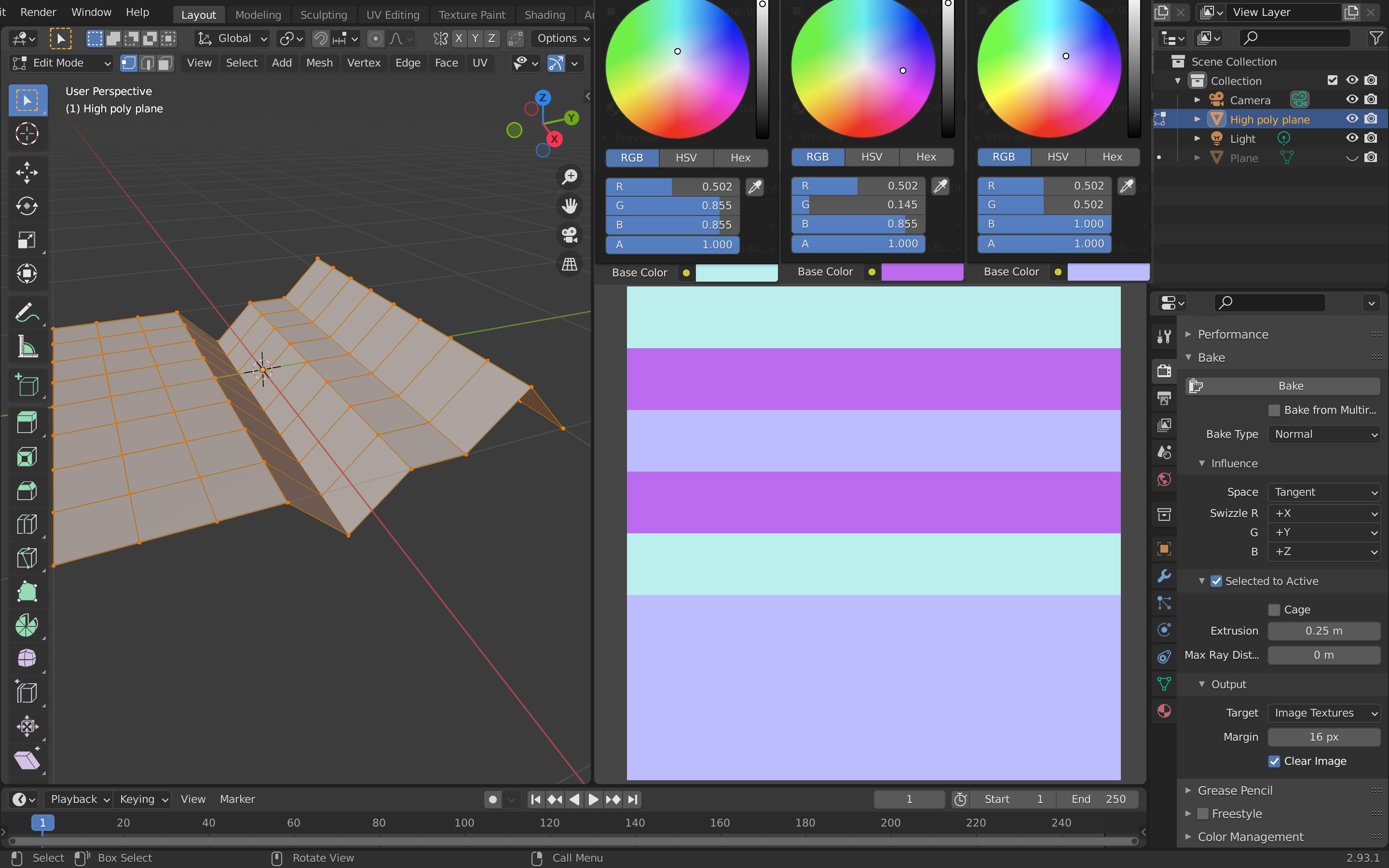In the last post I used a method called Reoriented Normal Mapping(RNP) to combine two normal maps. But I didn’t know what I was doing, so I spend some time to figure it out.

## Normal map

Fist I need to know what is a normal map, I found this Unity manual explains very well.

I baked a simple normal map to a two-meter by two-meter plane, the angle of the slope is 45 degrees. The normals are $$(0, {\sqrt 2 \over 2}, {\sqrt 2 \over 2})$$, $$(0, - {\sqrt 2 \over 2}, {\sqrt 2 \over 2})$$ and $$(0, 0, 1)$$. Values of the unit vector are in the range of [-1, 1], convert them to [0, 1] by add one then divide by two: $$(0.5, {\sqrt 2 \over 4} + {1 \over 2} \approx 0.854, 0.854)$$, $$(0.5, - {\sqrt 2 \over 4} + {1 \over 2} \approx 0.146, 0.854)$$, $$(0.5, 0.5, 1)$$Blender casts rays from the low-poly object inwards towards the high-poly object while baking therefore the Extrusion parameter should be at least $${2 \over 8} = 0.25m$$ in order to bake the protruding part. And the normal map image node’s Color Space must be set to Non-Color, otherwise the Image Editor will display the normal map as an ordinary image.

## Quaternion

Next I need to know what the heck is quaternion and why half angles are used in 3D rotation. The amazing website created by Grant Sanderson and Ben Eater is really helpful for me to understand the concept.

## Reoriented Normal Mapping

I made a video to demonstrate how RNP combines two normal vectors by rotating vector :

Let $$\color{#83C167}{\boldsymbol{u}}$$ be the first normal vector and $$\color{#58C4DD}{\boldsymbol{s}}$$ is $$\color{#58C4DD}{(0, 0, 1)}$$, $$\color{#FC6255}{\boldsymbol{t}}$$ is the second normal vector, $$\color{#D147BD}{\boldsymbol{r}}$$ is the reoriented normal and $$\theta$$ is the angle between $$\color{#58C4DD}{\boldsymbol{s}}$$ and $$\color{#FC6255}{\boldsymbol{t}}$$. By finding the quaternion $$\boldsymbol{q}$$ which rotates $$\color{#58C4DD}{\boldsymbol{s}}$$ to $$\color{#FC6255}{\boldsymbol{t}}$$, we can then use it to rotate $$\color{#83C167}{\boldsymbol{u}}$$ to get $$\color{#D147BD}{\boldsymbol{r}}$$. The length of the imaginary part of $$\boldsymbol{q}$$ is $$\sin({\theta \over 2})$$ and the length of the real part is $$\cos({\theta \over 2})$$.

The orientation of the imaginary(vector) part of $$\boldsymbol{q}$$ can be obtained by applying the right hand rule on $$\boldsymbol{s}$$ and $$\boldsymbol{t}$$, that’s the same orientation of $$\boldsymbol{s} \times \boldsymbol{t}$$. Recall the length of cross product of two unit vectors is sine of the angles between them. Therefore the imaginary part of $$\boldsymbol{q}$$ is:

$\boldsymbol{q_v} = \boldsymbol{s} \times \boldsymbol{t} {\sin {\theta \over 2} \over \sin \theta}$

Using the Pythagorean identity:

$\sin^2\theta + \cos^2 \theta = 1$

and the double-angle formulae:

$\cos(2\theta) = 1 - 2\sin^2 \theta = 2\cos^2 \theta - 1$

$\boldsymbol{q_v} = \boldsymbol{s} \times \boldsymbol{t} \sqrt {1 - \cos \theta \over 2 (1 - \cos^2 \theta)} = {\boldsymbol{s} \times \boldsymbol{t} \over \sqrt {2 (1 + \cos \theta)}}$

Recall the dot product of two unit vectors is the cosine of the angle between them:

$\boldsymbol{q_v} = {\boldsymbol{s} \times \boldsymbol{t} \over \sqrt {2 (1 + \boldsymbol{s} \cdot \boldsymbol{t})}}$

and the real part is:

$q_w = \cos {\theta \over 2} = \sqrt {1 + \cos \theta \over 2} = \sqrt {1 + \boldsymbol{s} \cdot \boldsymbol{t} \over 2}$

Use quaternions to transform $$\boldsymbol{u}$$ to $$\boldsymbol{r}$$:

$\boldsymbol{r} = \boldsymbol{q} \boldsymbol{p} \boldsymbol{q^{-1}}$

$\boldsymbol{p} = (\boldsymbol{u}, 0)$

$\boldsymbol{q^{-1}} = (-\boldsymbol{q_v}, q_w)$

Using the quaternions multiplication equation:

$\boldsymbol{q} \boldsymbol{r} = (\boldsymbol{q_v} \times \boldsymbol{r_v} + r_w \boldsymbol{q_v} + q_w \boldsymbol{r_v}, q_w r_w - \boldsymbol{q_v} \cdot \boldsymbol{r_v})$

$$\boldsymbol{r}$$ can be represented by:

$\boldsymbol{r} = (-\boldsymbol{q_v} \times \boldsymbol{u} \times \boldsymbol{q_v} + 2q_w\boldsymbol{q_v} \times \boldsymbol{u} + q_w^2 \boldsymbol{u} + (\boldsymbol{q_v} \cdot \boldsymbol{u}) \boldsymbol{q_v}, 0)$

Using the triple product expansion formula:

$\boldsymbol{a} \times (\boldsymbol{b} \times \boldsymbol{c}) = \boldsymbol{b}(\boldsymbol{a} \cdot \boldsymbol{c}) - \boldsymbol{c}(\boldsymbol{a} \cdot \boldsymbol{b})$

to replace the triple product in $$\boldsymbol{r}$$:

$\boldsymbol{r} = \boldsymbol{q_v}(\boldsymbol{q_v} \cdot \boldsymbol{u}) - \boldsymbol{u}(\boldsymbol{q_v} \cdot \boldsymbol{q_v}) + 2q_w\boldsymbol{q_v} \times \boldsymbol{u} + q_w^2 \boldsymbol{u} + (\boldsymbol{q_v} \cdot \boldsymbol{u}) \boldsymbol{q_v}$

$= \boldsymbol{u}(q_w^2 - \boldsymbol{q_v} \cdot \boldsymbol{q_v}) + 2q_w\boldsymbol{q_v} \times \boldsymbol{u} + 2 \boldsymbol{q_v}(\boldsymbol{q_v} \cdot \boldsymbol{u})$

Rest steps can be found at the appendix of Self Shadow blog.

## Okay move along, move along people, there’s nothing to see here!

Here is the code that creates the RNP video: